# 1. Find the volume of the solid created by revolving the region bounded by the curves...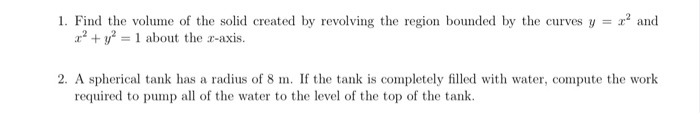1. Find the volume of the solid created by revolving the region bounded by the curves y = r2 and 2.2 + y2 = 1 about the 2-axis. 2. A spherical tank has a radius of 8 m. If the tank is completely filled with water, compute the work required to pump all of the water to the level of the top of the tank.

See dear these all are different lengthy problems. According to chegg guidelines I have to solve only the first question when multiple questions are given. So I am solving first question. Hope similarly you can solve other questions.Rate it.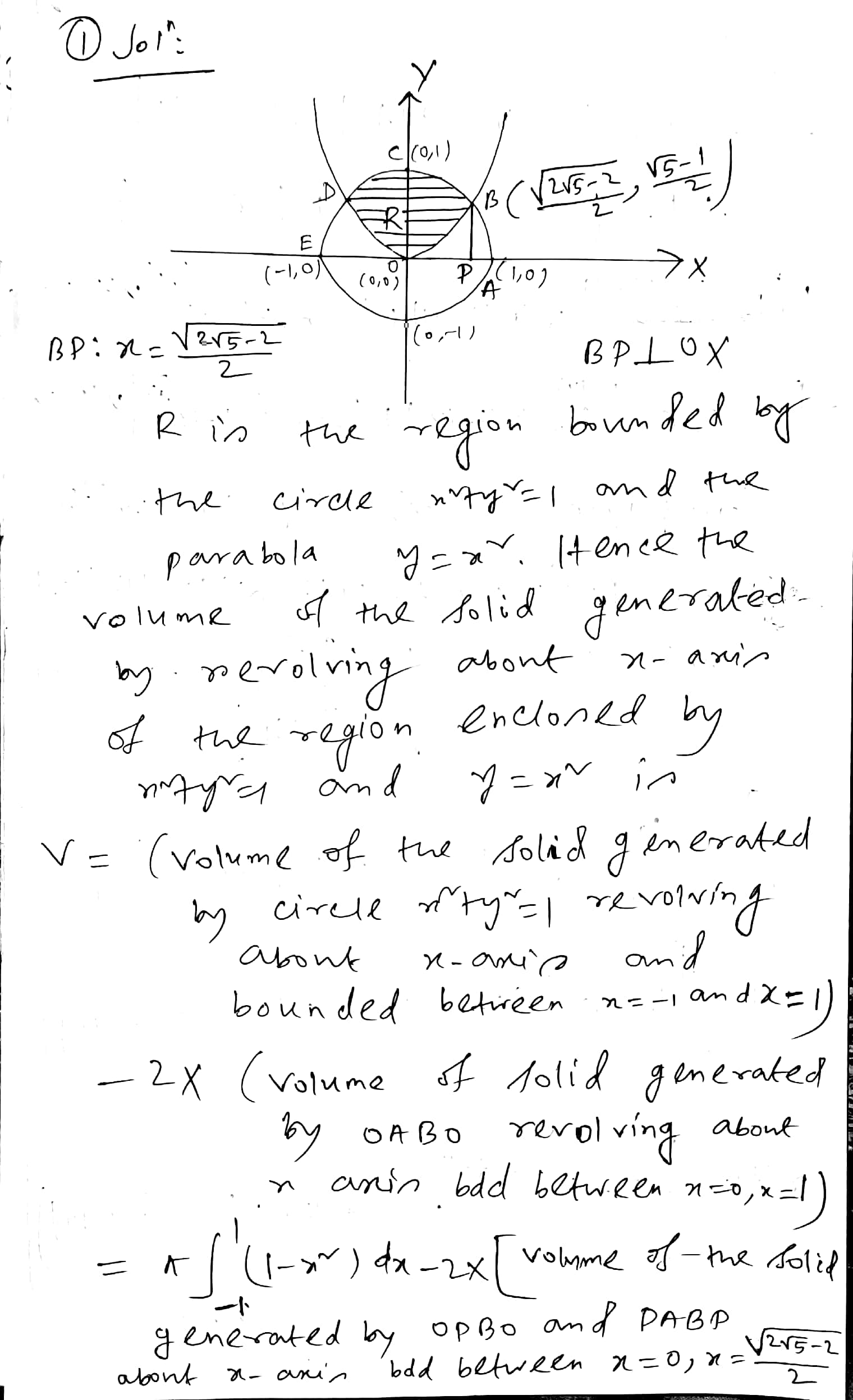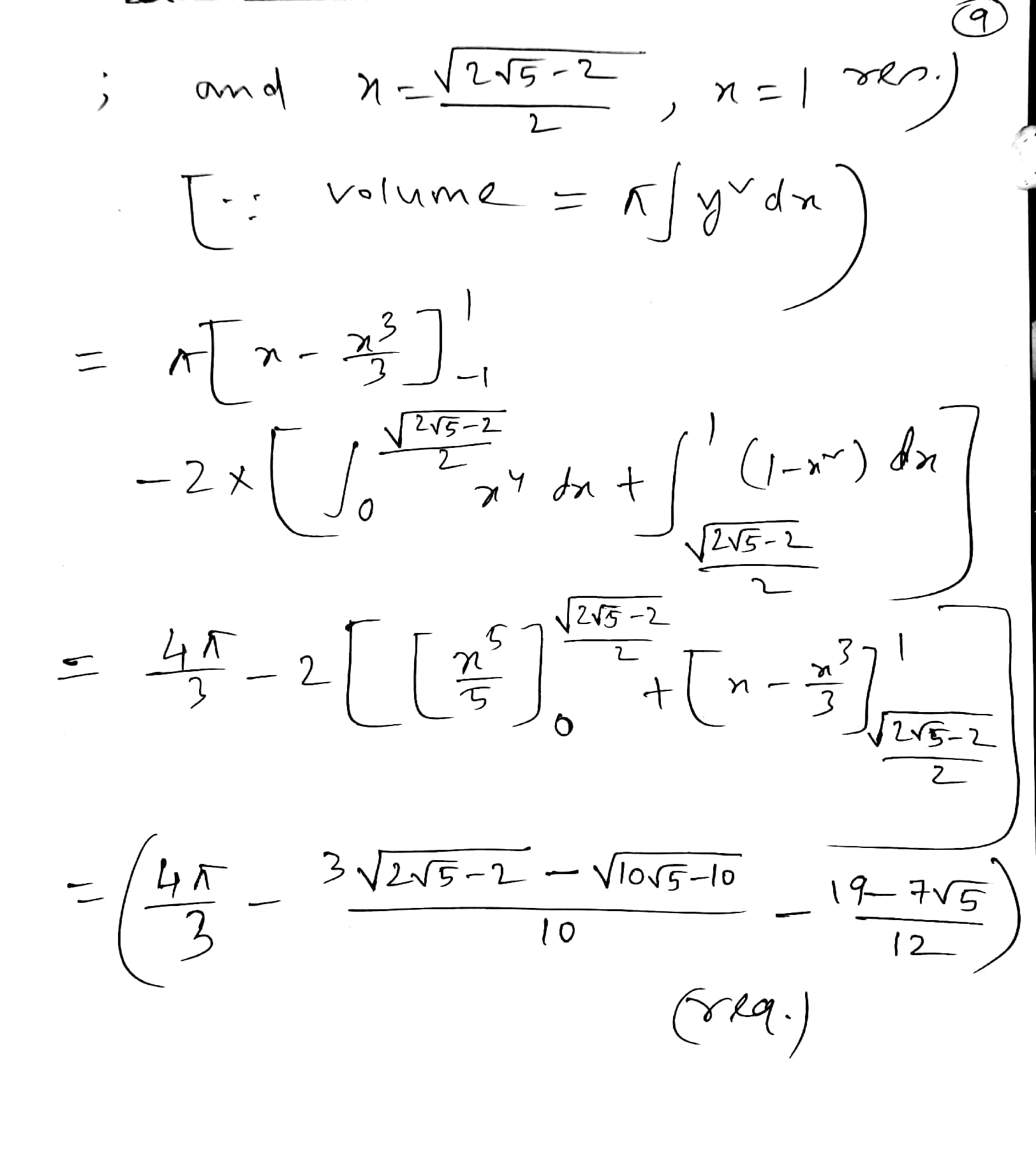##### Add Answer of: 1. Find the volume of the solid created by revolving the region bounded by the curves...
Similar Homework Help Questions
• ### Find the volume of the solid created by revolving the region bounded by the curves y=x2...

Find the volume of the solid created by revolving the region bounded by the curves y=x2 and x2+y2= 1 about the x-axis.

• ### 4. Find the volume of the solid generated by revolving the region bounded by the given...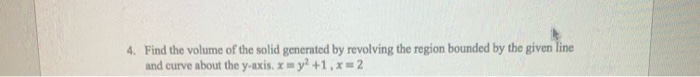4. Find the volume of the solid generated by revolving the region bounded by the given line and curve about the y-axis. x + y2 +1, x = 2

• ### 30) In order to find the volume of the solid of revolution created by revolving the area bounded by the curves y 4-...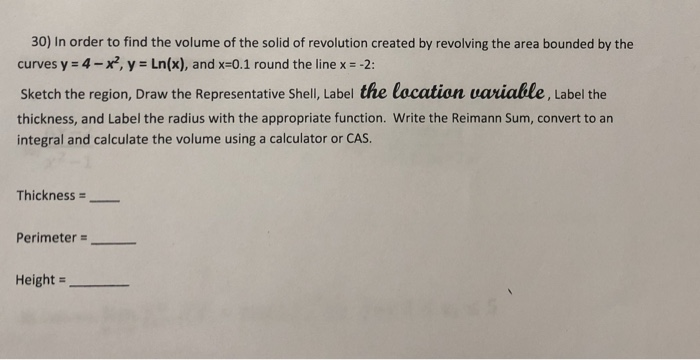30) In order to find the volume of the solid of revolution created by revolving the area bounded by the curves y 4-x2, y Ln(x), and x-0.1 round the line x= -2: Sketch the region, Draw the Representative Shell, Label the location variable, Label the thickness, and Label the radius with the appropriate function. Write the Reimann Sum, convert to an integral and calculate the volume using a calculator or CAS Thickness Perimeter Height = 30) In order to find...

• ### Sketch the region bounded by the following curves and find the volume of the solid generated by...

sketch the region bounded by the following curves and find the volume of the solid generated by revolving this region about the y-axis. x=1/2y^3x=4and y=0

• ### (a) Find the volume of the solid generated by revolving the region bounded by the graphs...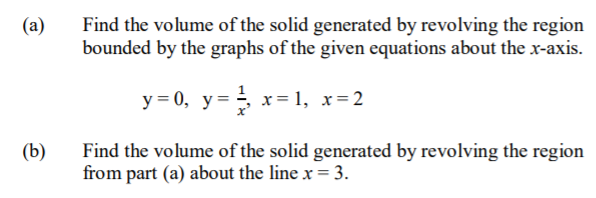(a) Find the volume of the solid generated by revolving the region bounded by the graphs of the given equations about the x-axis. y = 0, y= x= 1, x=2 (b) Find the volume of the solid generated by revolving the region from part (a) about the line x = 3.

• ### 1. (20 points) Find the volume of the solid formed by revolving the region bounded by...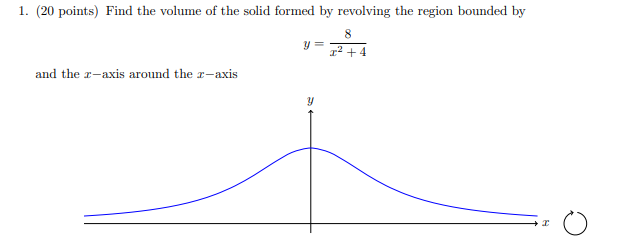1. (20 points) Find the volume of the solid formed by revolving the region bounded by 8 r2 + 4 and the z-axis around the c-axis y = y 2

• ### Find the volume of the solid generated by revolving the region bounded by the given lines...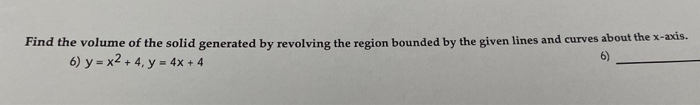Find the volume of the solid generated by revolving the region bounded by the given lines and curves about the x-axis. 6) y = x2 + 4, y = 4x + 4 6)

• ### b) Find the volume of the solid generated by revolving the region bounded on the left...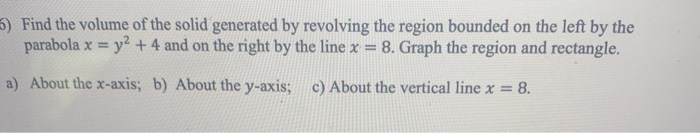b) Find the volume of the solid generated by revolving the region bounded on the left by the parabola x = y2 + 4 and on the right by the line x = 8. Graph the region and rectangle. a) About the x-axis; b) About the y-axis; c) About the vertical line x = 8.

• ### 6) Find the volume of the solid generated by revolving the region bounded on the left...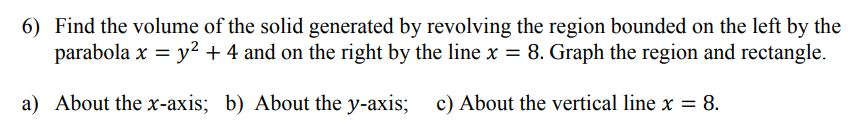6) Find the volume of the solid generated by revolving the region bounded on the left by the parabola x = y2 + 4 and on the right by the line x = 8. Graph the region and rectangle. a) About the x-axis; b) About the y-axis; c) About the vertical line x = 8.

• ### Find the volume of the solid formed by revolving the region bounded by

Find the volume of the solid formed by revolving the region bounded by y=(√x), y=1 and x=4 about the x-axis. Sketch graph.

Free Homework App